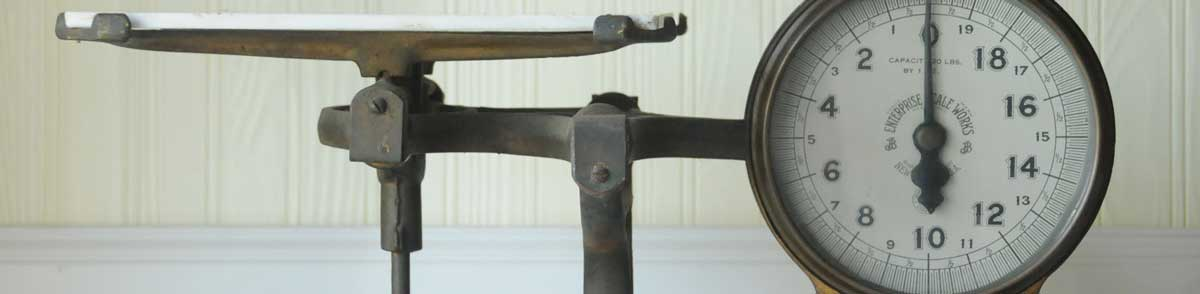# Ounces to Long Tons Conversion

Enter the weight in ounces below to get the value converted to long tons.

Results in Long Tons:1 oz = 2.7902E-5 lt
Hint: use a scientific notation calculator to convert E notation to decimal
Do you want to convert long tons to ounces?

## How to Convert Ounces to Long TonsTo convert an ounce measurement to a long ton measurement, multiply the weight by the conversion ratio.

Since one ounce is equal to 2.7902E-5 long tons, you can use this simple formula to convert:

long tons = ounces × 2.7902E-5

The weight in long tons is equal to the ounces multiplied by 2.7902E-5.

For example, here's how to convert 50,000 ounces to long tons using the formula above.
50,000 oz = (50,000 × 2.7902E-5) = 1.395089 lt

## Ounces

One ounce is a unit of mass/weight equal to 1/16 of a pound. The common ounce should not be confused with the troy ounce, which is equal to 1/12 of a troy pound.

The ounce is a US customary and imperial unit of weight. Ounces can be abbreviated as oz; for example, 1 ounce can be written as 1 oz.

## Long Tons

One long ton is equal to 2,240 pounds and is mostly used in the United Kingdom as a unit of mass/weight. Long tons are not the same as the short ton, which is used mostly in the United States, or the metric ton which is used throughout the world.

The long ton is an imperial unit of weight. A long ton is sometimes also referred to as an imperial ton or displacement ton. Long tons can be abbreviated as lt, and are also sometimes abbreviated as t. For example, 1 long ton can be written as 1 lt or 1 t.

## Ounce to Long Ton Conversion Table

Ounce measurements converted to long tons
Ounces Long Tons
1 oz 0.000027902 lt
2 oz 0.000055804 lt
3 oz 0.000083705 lt
4 oz 0.000112 lt
5 oz 0.00014 lt
6 oz 0.000167 lt
7 oz 0.000195 lt
8 oz 0.000223 lt
9 oz 0.000251 lt
10 oz 0.000279 lt
100 oz 0.00279 lt
1,000 oz 0.027902 lt
10,000 oz 0.279018 lt
100,000 oz 2.7902 lt

## References

1. James Bucki, Troy Ounce vs. Ounce, The Spruce, https://www.thesprucecrafts.com/troy-ounce-vs-ounce-4588158
2. State of California Division of Measurement Standards, Brief History of Weights and Measures, https://www.cdfa.ca.gov/dms/trainingmodules/Module_05.pdf